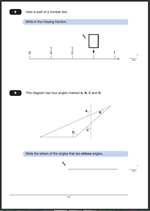# Resource of the Week: Maths SAT questionsFor anyone in Year 6 the Maths SAT tests will be coming up before too long! Don’t forget that we have a whole section of past questions and answers, including great explanations of the best ways to approach each question. Invaluable help to achieve those higher levels!

This week I am highlighting are two questions from the Maths SAT Paper 2011 Paper B. The first involves equivalent fractions and the second angles and shape.

The answer to Question 8.   3/4
One mark awarded for a correct answer.
Equivalent fractions accepted as is 0.75.
Suggested method:
This question is another reading a number line problem and if children can count in quarters it is an easy mark. Firstly, it has to be recognised that the number line is showing quarters (1/2 is equivalent to 2/4 and 1 is equivalent to 4/4) making the missing number 3/4.

The answer to Question 9. A and D.
One mark awarded for a correct answer.
The letters can be given in either order. Both need to be given with no incorrect angles added.
Suggested method:
Answering this question correctly depends on 3 things:
Firstly, a recognition of the conventions for labelling angles, using the arc.
Secondly, a knowledge that an obtuse angle is larger than 90 degrees but less than 180 degrees.
If either of these are unknown it becomes purely a guessing game. (Some children do think that the angle depends on the length of the lines rather than a measure of turn.)

Thirdly, if these are known, then the obtuse angles still need to be recognised. One way to do this is to slide a right angle (eg a corner of a piece of paper) into the angle to see whether it is smaller or larger.

Why not visit ks2-maths-sats.co.uk for free SATs papers and a great SAT revision programme?

Questions 8 and 9 from SAT Paper B 2011

Questions 8 and 9 answers and suggested method 2011 Paper B